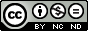### Note on the conformable boundary value problems: Sturm’s theorems and Green’s function

F. Martínez, I. Martínez, M. K. A. Kaabar, S. Paredes

#### Abstract

Recently, the conformable derivative and its properties have been introduced. In this paper, we propose and prove some new results on conformable Boundary Value Problems. First, we introduce a conformable version of classical Sturm´s separation, and comparison theorems. For a conformable Sturm-Liouville problem, Green's function is constructed, and its properties are also studied. In addition, we propose the applicability of the Green´s Function in solving conformable inhomogeneous linear differential equations with homogeneous boundary conditions, whose associated homogeneous boundary value problem has only trivial solution. Finally, we prove the generalized Hyers-Ulam stability of the conformable inhomogeneous boundary value problem.

#### Keywords

Conformable fractional derivative; Conformable fractional integral; Conformable fractional differential equations; Sturm´s Theorems; Green´s Function

PDF

#### References

A. Kilbas, H. Srivastava, J. Trujillo, (2006) Theory and Applications of Fractional Differential Equations; North-Holland, New York.

K. S. Miller, (1993) An Introduction to Fractional Calculus and Fractional Differential Equations; J. Wiley and Sons, New York.

R. Khalil, M. Al Horani, A. Yousef, M. A. Sababheh, New definition of fractional derivative. J. Comp. Appl. Math., 264 (2014), 65–70.

T. Abdeljawad, On conformable fractional calculus. J. Comp. Appl. Math., 279 (2015), 57–66.

O. S. Iyiola, E. R. Nwaeze, Some new results on the new conformable fractional calculus with application using D´Alambert approach. Progr. Fract. Differ. Appl., 2 (2016), 1–7.

A. Atangana, D. Baleanu, A. Alsaedi, New properties of conformable derivative. Open Math., 13 (2015), 57–63.

N. Yazici, U. Gözütok, Multivariable Conformable Fractional Calculus. Filomat, 32 (2018), 45-53.

F. Martínez, I. Martínez, S. Paredes, Conformable Euler´s Theorem on homogeneous functions. Comp. and Math. Methods, (2018), 1–11.

E. Ünal, A. Gökdogan, E. Çelik, Solutions of Sequential Conformable Fractional Differential Equations around an Ordinary Point and Conformable Fractional Hermite Differential Equation, British Journal of Applied Science & Technology, 10 (2015), 1-11.

M. Al Masalmeh, Series Method to solve conformable fractional Riccati Differential equations, International Journal of Applied Mathematics Research, 6 (2017), 30-33.

M. Al Horani, R. Khalil, Total fractional differential with applications to exact fractional differential equations, International Journal of Computer Mathematics, 95 (2018), 1444–1452.

M. Horani, M. A. Hammad, R. Khalil, Variations of parameters for local fractional nonhomogeneous linear-differential equations, J. Math. Computer Sci., 16 (2016), 147–153.

R. Khalil, M. A. Al Horani, D. Anderson, Undetermined coefficients for local differential equations, J. Math. Computer Sci., 16 (2016), 140–146.

M. A. Hammad, R. Khalil, Abel´s formula and wronskian for conformable fractional differential equations, International Journal of Differential Equations and Applications, 13 (2014), 177–183.

M. A. Hammad, R. Khalil, Legendre fractional differential equation and Legendre fractional polynomials, International Journal of Applied Mathematical Research, 3 (2014), 214–219.

F. S. Silva, M. D. Moreira, M. A. Moret, Conformable Laplace Transform of Fractional Differential Equations. Axioms, 7 (2018), 1–12.

Z. Al-Zhour, N. Al-Mutairi, F. Alrawajeh, R. Alkhasawneh, Series solutions for the Laguerre and Lane-Emden fractional differential equations in the sense of conformable fractional derivative. Alexandria Engineering Journal, 58 (2019), 1413–1420.

M. A. Hammad, H. Alzaareer, H. Al-Zoubi, H. Dutta, Fractional Gauss hypergeometric differential equation, Journal of Interdisciplinary Mathematics, 22 (2019), 1113–1121.

W. R. Derrick, S. I. Grossman, (1981) Elementary Differential Equations with Applications; Addison-Wesley Publising Company, Inc., de Reading.

C. T. Fulton, L. Wu, S. Pruess, A Sturm Separation Theorem for linear 2nth order Self-adjoint Differential Equation, Journal applied Mathematics and Stochastic Analysis, 8 (1995), 29-46.

M. Kaabar, Novel Methods for Solving the Conformable Wave Equation. Journal of New Theory, 31 (2020), 56–85.

G. Choi, S. M. Jung, Invariance of Hyers-Ulam stability of linear differential equations and its applications. Advances in Differential equations, 2015 (2015), 1-14.

F. Martínez, I. Martínez, M. K. A. Kaabar, R. Ortíz-Munuera, S. Paredes, Note on the Conformable Fractional Derivatives and Integrals of Complex-Valued Functions of a Real Variable. IAENG International Journal of Applied Mathematics, 50 (2020), 609–615.

F. Martínez, I. Martínez, M. K. A. Kaabar, S. Paredes, New results on complex conformable integral. AIMS Mathematics, 5 (2020), 7695-7710.

DOI: https://doi.org/10.31349/RevMexFis.67.471

### Refbacks

• There are currently no refbacks.